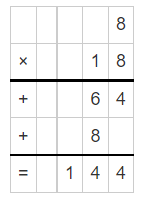# McGraw Hill Math Grade 4 Chapter 5 Lesson 4 Answer Key Multiplying a Two-Digit Number by a Two-Digit Number

Practice the questions of McGraw Hill Math Grade 4 Answer Key PDF Chapter 5 Lesson 4 Multiplying a Two-Digit Number by a Two-Digit Number to secure good marks & knowledge in the exams.

## McGraw-Hill Math Grade 4 Answer Key Chapter 5 Lesson 4 Multiplying a Two-Digit Number by a Two-Digit Number

Multiply

Find each product. On a separate sheet of paper, draw arrays to show partial products.

Question 1.
15 × 19 = 285
Explanation: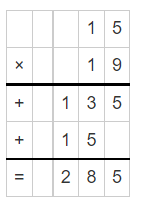Question 2.
22 × 17 = ____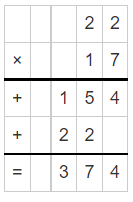Explanation:
The product of 22 and 17 is 374
take the carried value and place it in the column to the left.
So if you were multiplying numbers in the tens column and you needed to carry a number,
you would place that “number” in the hundreds column.

Question 3.
53 × 26 = ____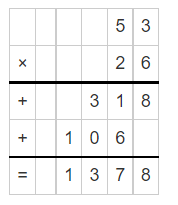Explanation:
The product of  53 and 26 is 1378
take the carried value and place it in the column to the left.
So if you were multiplying numbers in the tens column and you needed to carry a number,
you would place that “number” in the hundreds column.

Question 4.
11 × 14 = ___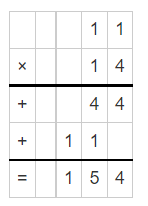Explanation:
The product of 11 and 14 is 154
take the carried value and place it in the column to the left.
So if you were multiplying numbers in the tens column and you needed to carry a number,
you would place that “number” in the hundreds column.

Question 5.
25 × 12 = ___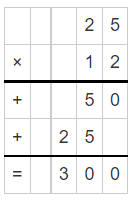Explanation:
The product of 25 and 12 is 300
take the carried value and place it in the column to the left.
So if you were multiplying numbers in the tens column and you needed to carry a number,
you would place that “number” in the hundreds column.

Question 6.
8 × 18 = ___